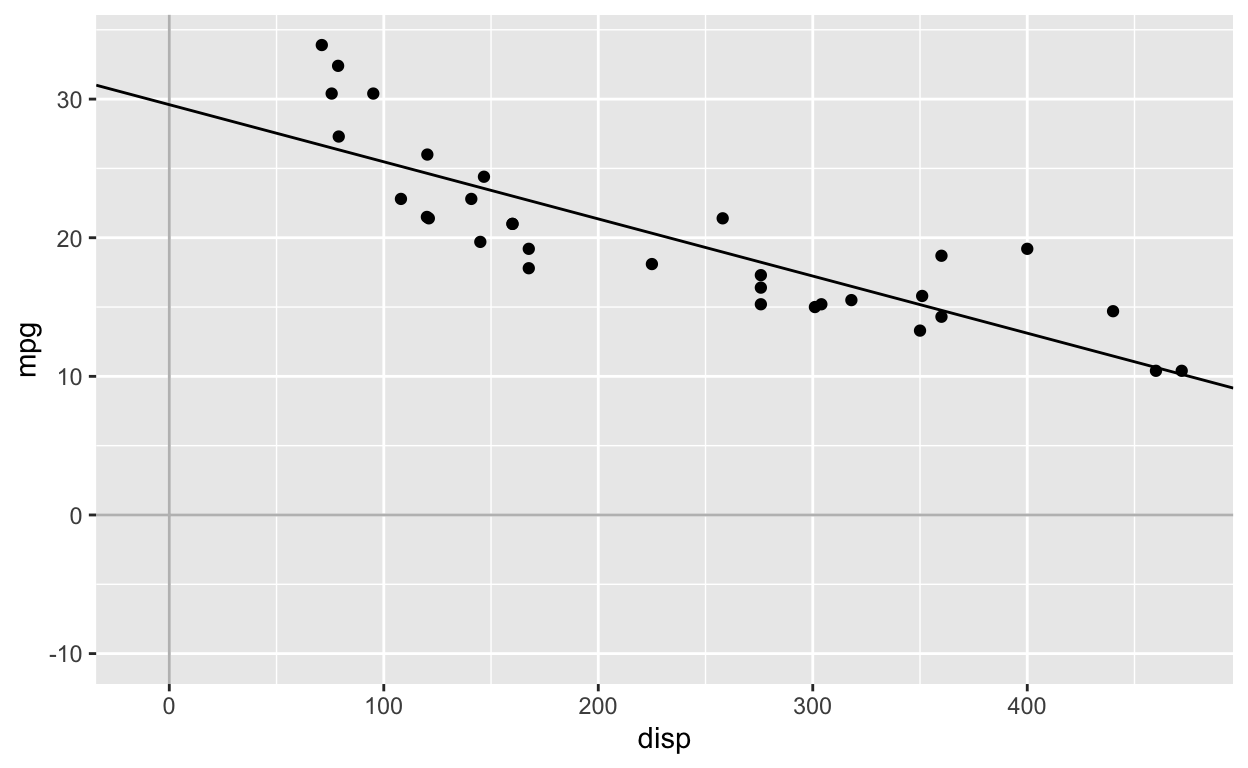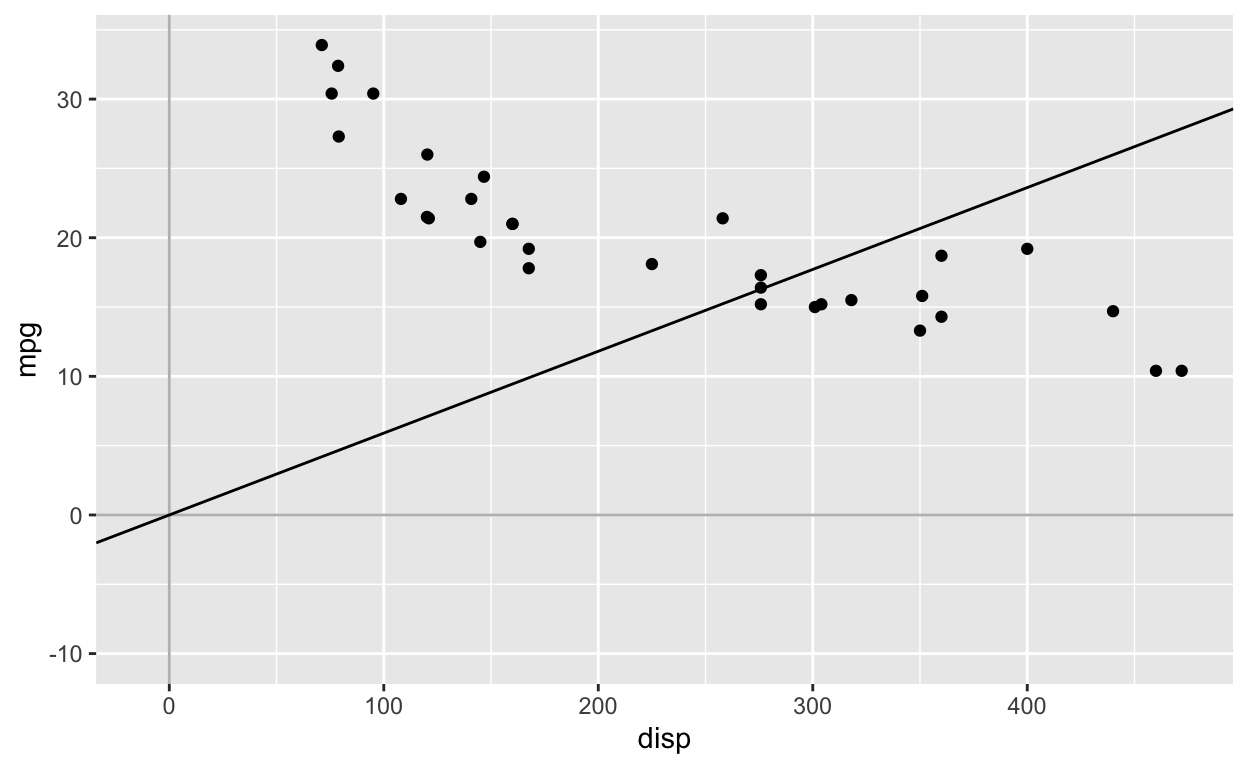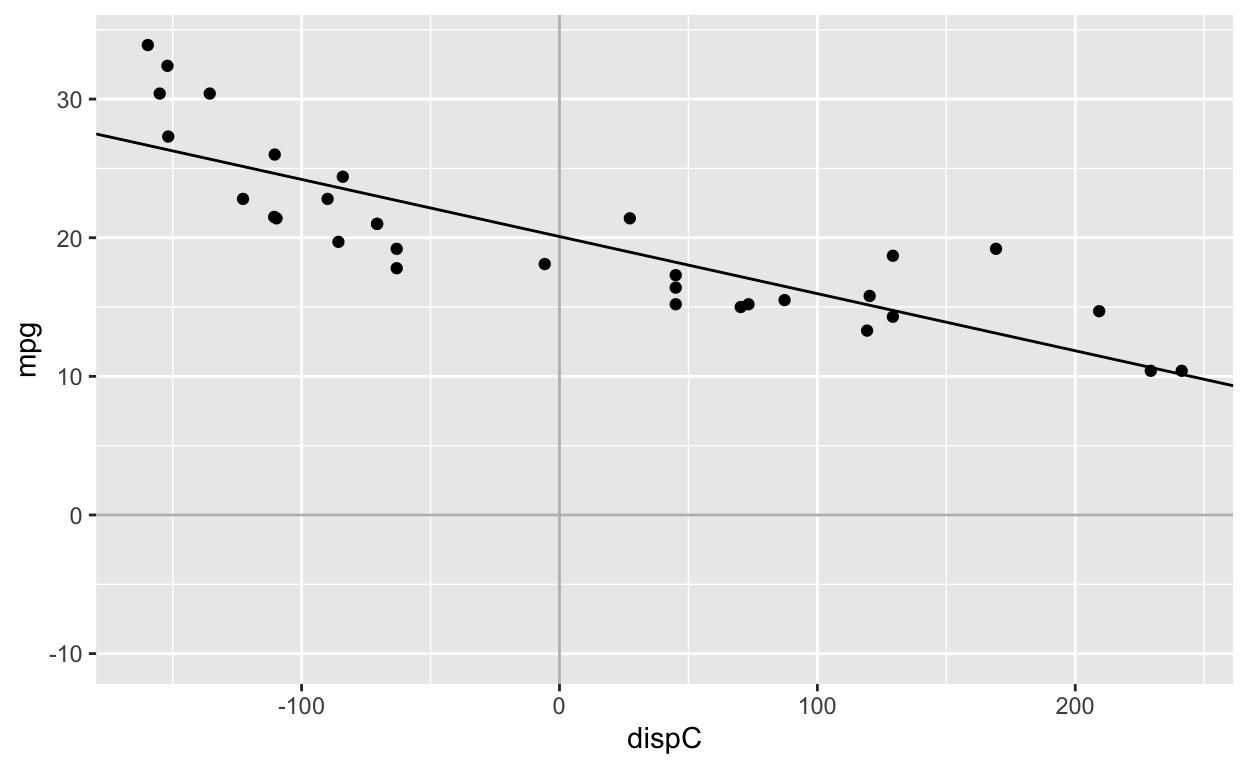# What happens when you set the intercept to 0 in regression models

What happens when you force the intercept to be 0 in a regression model and why you should (generally) never do it

Hause Lin true
07-24-2019

Get source code for this RMarkdown script here.

## Consider being a patron and supporting my work?

Donate and become a patron: If you find value in what I do and have learned something from my site, please consider becoming a patron. It takes me many hours to research, learn, and put together tutorials. Your support really matters.

This article answers the questions below. I will use the built-in dataset mtcars.

• What is the intercept in a regression model?
• What happens when you remove or set the intercept to 0 in a regression model?
• Why you should never remove or set the intercept to 0?
• What are the effects of mean-centering a regressor/predictor?

If you need a refresher on how to interpret regression coefficients, see my other article.


library(ggplot2) # plot regression lines

Have a look at the built-in mtcars dataset.


head(mtcars)

mpg cyl disp  hp drat    wt  qsec vs am gear carb
Mazda RX4         21.0   6  160 110 3.90 2.620 16.46  0  1    4    4
Mazda RX4 Wag     21.0   6  160 110 3.90 2.875 17.02  0  1    4    4
Datsun 710        22.8   4  108  93 3.85 2.320 18.61  1  1    4    1
Hornet 4 Drive    21.4   6  258 110 3.08 3.215 19.44  1  0    3    1
Hornet Sportabout 18.7   8  360 175 3.15 3.440 17.02  0  0    3    2
Valiant           18.1   6  225 105 2.76 3.460 20.22  1  0    3    1

## Fit simple regression model (with intercept)


model1 <- lm(mpg ~ disp, mtcars) # the intercept is included by default: lm(mpg ~ 1 + disp, mtcars)
coef1 <- coef(model1) # get coefficients
coef1

(Intercept)        disp
29.59985476 -0.04121512 

Regression equation:

$mpg_{i} = 29.59 - 0.04*disp_{i}$

Interpretation

• For every 1 unit increase in the predictor disp, the outcome mpg changes by -0.04. That is, as disp increases, mpg decreases.
• When disp = 0, mpg = 29.59.

$mpg = 29.59 - 0.04*0$

$mpg = 29.59$


ggplot(mtcars, aes(disp, mpg)) +
geom_point() +
geom_vline(xintercept = 0, col = 'grey') +
geom_hline(yintercept = 0, col = 'grey') +
scale_x_continuous(limits = c(-10, max(mtcars$disp))) + scale_y_continuous(limits = c(-10, max(mtcars$mpg))) +
geom_abline(intercept = coef(model1), slope = coef(model1)) # manually plot regression line## Fit simple regression model without intercept


model0 <- lm(mpg ~ 0 + disp, mtcars) # equivalent syntax: lm(mpg ~ -1 + disp, mtcars)
coef0 <- coef(model0) # get coefficients
coef0

disp
0.05904912 

Note that after setting the intercept to 0, the relationship between mpg and disp is now POSITIVE, rather than negative (see above model with intercept).

Regression equation:

$mpg_{i} = 0 + 0.059*disp_{i}$

Interpretation

• For every 1 unit increase in the predictor disp, the outcome mpg changes by 0.059. That is, as disp increases, mpg increases.
• When disp = 0, mpg = 0. By removing the intercept (i.e., setting it to 0), we are forcing the regression line to go through the origin (the point where disp = 0 and mpg = 0).

$mpg = 0 + 0.059*0$

$mpg = 0$

The regression line is forced to pass through the origin (0, 0). Therefore, unless your regressors are standardized or mean-centered, it’s not a good idea to set the intercept to 0 when fitting the model. Even when your regressors are standardized or mean-centered, you should still include the intercept.


ggplot(mtcars, aes(disp, mpg)) +
geom_point() +
geom_vline(xintercept = 0, col = 'grey') +
geom_hline(yintercept = 0, col = 'grey') +
scale_x_continuous(limits = c(-10, max(mtcars$disp))) + scale_y_continuous(limits = c(-10, max(mtcars$mpg))) +
geom_abline(intercept = 0, slope = coef(model0)) # manually plot regression line## Fit simple regression models with mean-centered regressor

Mean-center regressor


mtcars$dispC <- mtcars$disp - mean(mtcars$disp) # create mean-centered variable mean(mtcars$dispC) # mean of dispC is 0 (with some rounding error)

 -1.199041e-14

Fit model with intercept and mean-centered regressor


model1c <- lm(mpg ~ dispC, mtcars)
coef(model1c)

(Intercept)       dispC
20.09062500 -0.04121512 

Fit model without intercept and with mean-centered regressor


model0c <- lm(mpg ~ 0 + dispC, mtcars)
coef(model0c)

dispC
-0.04121512 

After mean-centering the regressor/predictor, fitting the model with or without the intercept gives the same dispC coefficient: -0.04


ggplot(mtcars, aes(dispC, mpg)) +
geom_point() +
geom_vline(xintercept = 0, col = 'grey') +
geom_hline(yintercept = 0, col = 'grey') +
scale_x_continuous(limits = c(min(mtcars$dispC), max(mtcars$dispC))) +
scale_y_continuous(limits = c(-10, max(mtcars\$mpg))) +
geom_abline(intercept = coef(model1c), slope = coef(model1c))Note that the regression slope is identical to the first figure. The only difference is that the points have been shifted to the left.

## Support my work

### Corrections

If you see mistakes or want to suggest changes, please create an issue on the source repository.

### Reuse

Text and figures are licensed under Creative Commons Attribution CC BY 4.0. Source code is available at https://raw.githubusercontent.com/hauselin/rtutorialsite/master/_posts/2019-07-24-what-happens-when-you-remove-or-set-the-intercept-to-0-in-regression-models/what-happens-when-you-remove-or-set-the-intercept-to-0-in-regression-models.Rmd, unless otherwise noted. The figures that have been reused from other sources don't fall under this license and can be recognized by a note in their caption: "Figure from ...".

### Citation

Lin (2019, July 24). Data science: What happens when you set the intercept to 0 in regression models. Retrieved from https://hausetutorials.netlify.com/posts/2019-07-24-what-happens-when-you-remove-or-set-the-intercept-to-0-in-regression-models/
@misc{lin2019what,
}